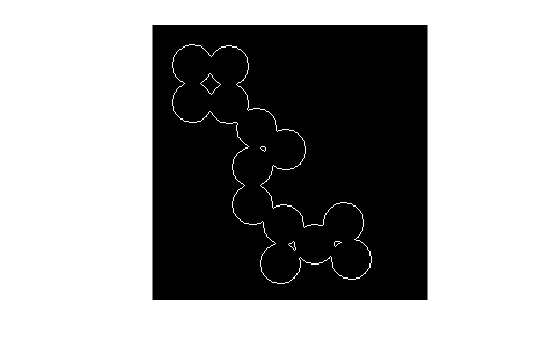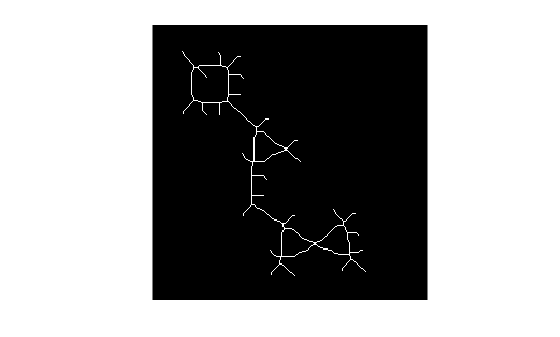# bwmorph

Morphological operations on binary images

## Syntax

``BW2 = bwmorph(BW,operation)``
``BW2 = bwmorph(BW,operation,n)``

## Description

example

````BW2 = bwmorph(BW,operation)` applies a specific morphological operation to the binary image `BW`. NoteTo perform morphological operations on a 3-D volumetric image, use `bwmorph3`. ```
````BW2 = bwmorph(BW,operation,n)` applies the operation `n` times. `n` can be `Inf`, in which case the operation is repeated until the image no longer changes.```

## Examples

collapse all

Read binary image and display it.

```BW = imread('circles.png'); imshow(BW);```Remove interior pixels to leave an outline of the shapes.

```BW2 = bwmorph(BW,'remove'); figure imshow(BW2)```Get the image skeleton.

```BW3 = bwmorph(BW,'skel',Inf); figure imshow(BW3)```## Input Arguments

collapse all

Binary image, specified as a 2-D numeric matrix or 2-D logical matrix. For numeric input, any nonzero pixels are considered to be `1` (`true`).

Data Types: `single` | `double` | `int8` | `int16` | `int32` | `int64` | `uint8` | `uint16` | `uint32` | `uint64` | `logical`

Morphological operation to perform, specified as one of the following.

Operation

Description

`'bothat'`

Perform the morphological bottom hat operation, returning the image minus the morphological closing of the image.

The `bwmorph` function performs morphological closing using the neighborhood `ones(3)`. If you want to perform a morphological bottom hat operation with a different neighborhood, then use the `imbothat` function.

`'branchpoints'`

Find branch points of skeleton. For example:

```0 0 1 0 0 0 0 0 0 0 0 0 1 0 0 0 0 0 0 0 1 1 1 1 1 becomes 0 0 1 0 0 0 0 1 0 0 0 0 0 0 0 0 0 1 0 0 0 0 0 0 0```

Note: To find branch points, the image must be skeletonized. To create a skeletonized image, use `bwmorph(BW,'skel')`.

`'bridge'`

Bridge unconnected pixels, that is, sets `0`-valued pixels to `1` if they have two nonzero neighbors that are not connected. For example:

```1 0 0 1 1 0 1 0 1 becomes 1 1 1 0 0 1 0 1 1 ```

`'clean'`

Remove isolated pixels (individual `1`s that are surrounded by `0`s), such as the center pixel in this pattern.

```0 0 0 0 1 0 0 0 0 ```

`'close'`

Perform morphological closing (dilation followed by erosion).

The `bwmorph` function performs morphological closing using the neighborhood `ones(3)`. If you want to perform a morphological closing operation with a different neighborhood, then use the `imclose` function.

`'diag'`

Use diagonal fill to eliminate 8-connectivity of the background. For example:

```0 1 0 0 1 0 1 0 0 becomes 1 1 0 0 0 0 0 0 0 ```

`'endpoints'`

Find end points of skeleton. For example:

```1 0 0 0 1 0 0 0 0 1 0 0 becomes 0 0 0 0 0 0 1 0 0 0 1 0 0 0 0 0 0 0 0 0```

Note: To find end points, the image must be skeletonized. To create a skeletonized image, use `bwmorph(BW,'skel')`.

`'fill'`

Fill isolated interior pixels (individual `0`s that are surrounded by `1`s), such as the center pixel in this pattern.

```1 1 1 1 0 1 1 1 1 ```

`'hbreak'`

Remove H-connected pixels. For example:

```1 1 1 1 1 1 0 1 0 becomes 0 0 0 1 1 1 1 1 1 ```

`'majority'`

Set a pixel to `1` if five or more pixels in its 3-by-3 neighborhood are `1`; otherwise, set the pixel to `0`.

`'open'`

Perform morphological opening (erosion followed by dilation).

The `bwmorph` function performs morphological opening using the neighborhood `ones(3)`. If you want to perform a morphological opening operation with a different neighborhood, then use the `imopen` function.

`'remove'`

Remove interior pixels. This option sets a pixel to `0` if all its 4-connected neighbors are `1`, thus leaving only the boundary pixels on.

`'shrink'`

With `n = Inf`, shrink objects to points by removing pixels from the boundaries of objects. Objects without holes shrink to a point, and objects with holes shrink to a connected ring halfway between each hole and the outer boundary. This option preserves the Euler number (also known as the Euler characteristic).

`'skel'`

With `n = Inf`, remove pixels on the boundaries of objects without allowing objects to break apart. The pixels remaining make up the image skeleton. This option preserves the Euler number.

When working with 3-D volumes, or when you want to prune a skeleton, use the `bwskel` function.

`'spur'`

Remove spur pixels. For example:

```0 0 0 0 0 0 0 0 0 0 0 0 0 0 0 0 0 0 1 0 becomes 0 0 0 0 0 1 0 0 0 1 0 0 1 1 0 0 1 1 0 0 ```

`'thicken'`

With `n = Inf`, thicken objects by adding pixels to the exterior of objects until doing so would result in previously unconnected objects being 8-connected. This option preserves the Euler number.

`'thin'`

With `n = Inf`, thin objects to lines by removing pixels from the boundary of objects. An object without holes shrinks to a minimally connected stroke, and an object with holes shrinks to a connected ring halfway between each hole and the outer boundary. This option preserves the Euler number. See Algorithms for more detail.

`'tophat'`

Perform the morphological top hat operation, returning the image minus the morphological opening of the image.

The `bwmorph` function performs morphological opening using the neighborhood `ones(3)`. If you want to perform a morphological top hat operation with a different neighborhood, then use the `imtophat` function.

Tip

To perform morphological erosion or dilation, use the `imerode` or `imdilate` function, respectively. If you want to replicate the dilation or erosion performed by the `bwmorph` function, then specify the neighborhood as `ones(3)`.

Data Types: `char` | `string`

Number of times to perform the operation, specified as a positive integer or `Inf`. When you specify `n` as `Inf`, the `bwmorph` function repeats the operation until the image no longer changes.

Example: `100`

Data Types: `single` | `double` | `int8` | `int16` | `int32` | `int64` | `uint8` | `uint16` | `uint32` | `uint64`

## Output Arguments

collapse all

Image after morphological operations, returned as a 2-D logical matrix.

Data Types: `logical`

## Algorithms

collapse all

When used with the `'thin'` option, `bwmorph` uses the following algorithm :

1. In the first subiteration, delete pixel p if and only if the conditions G1, G2, and G3 are all satisfied.

2. In the second subiteration, delete pixel p if and only if the conditions G1, G2, and ${G}_{3}\prime$ are all satisfied.

### Condition G1:

`${X}_{H}\left(p\right)=1$`

where

`${X}_{H}\left(p\right)=\sum _{i=1}^{4}{b}_{i}$`

x1, x2, ..., x8 are the values of the eight neighbors of p, starting with the east neighbor and numbered in counter-clockwise order.

### Condition G2:

`$2\le \mathrm{min}\left\{{n}_{1}\left(p\right),{n}_{2}\left(p\right)\right\}\le 3$`

where

`${n}_{1}\left(p\right)=\sum _{k=1}^{4}{x}_{2k-1}\vee {x}_{2k}$`
`${n}_{2}\left(p\right)=\sum _{k=1}^{4}{x}_{2k}\vee {x}_{2k+1}$`

### Condition G3:

`$\left({x}_{2}\vee {x}_{3}\vee {\overline{x}}_{8}\right)\wedge {x}_{1}=0$`

### Condition G3':

`$\left({x}_{6}\vee {x}_{7}\vee {\overline{x}}_{4}\right)\wedge {x}_{5}=0$`

The two subiterations together make up one iteration of the thinning algorithm. When the user specifies an infinite number of iterations (`n=Inf`), the iterations are repeated until the image stops changing. The conditions are all tested using `applylut` with precomputed lookup tables.

 Haralick, Robert M., and Linda G. Shapiro, Computer and Robot Vision, Vol. 1, Addison-Wesley, 1992.

 Kong, T. Yung and Azriel Rosenfeld, Topological Algorithms for Digital Image Processing, Elsevier Science, Inc., 1996.

 Lam, L., Seong-Whan Lee, and Ching Y. Suen, "Thinning Methodologies-A Comprehensive Survey," IEEE Transactions on Pattern Analysis and Machine Intelligence, Vol 14, No. 9, September 1992, page 879, bottom of first column through top of second column.

 Pratt, William K., Digital Image Processing, John Wiley & Sons, Inc., 1991.